# Random Number Generators

If you just need code for above mentioned random number generators, you can find it here. However, I encourage you to read the theory behind them.

First of all, let’s have a look on the definition of all those terms written above.

1. Uniform Distribution: As the name suggests, distribution is uniform over the interval and zero everywhere else. It means that if I have a continuous interval ranging from [a,b] and I have to randomly pick an interval of length l, then probability of selection of [k, k + l] will be same as probability of selection of [j, j + l]. Assumption: a ≤ j, k, j + l, k + l ≤b.

2. Normal Distribution: Distribution over a given continuous interval is spread like a bell shaped curve. This will be more clear from the Figure 1.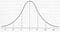Standard Normal distribution with mean = 0 and standard deviation = 1.

Note that in above case, if I have to choose a random interval, then probability that interval [0, 1] is chosen is more than probability that interval [1, 2] is chosen. Reason: Area under the curve in region [0, 1] is more than area under the curve [1, 2]. I will publish an article about various types of distributions soon. A more clear explanation can be found in that article.

3. Multivariate Standard Normal Distribution: It is almost similar to normal distribution. Only difference is that a standard normal distribution is in 1-D i.e. if you generate a data point from a standard normal distribution, then the generated data will have only one dimension, while in case of multivariate standard Normal distribution, your data can be of any dimension. This will be more clear from Figure 2.

In above Figure, for every (x, y) pair, there exists a value on Z-axis. Hence, if we sample a data point from this distribution, it will be of two dimensions. In laymen terms, each data point will contain two values. This concept can be extended to N-Dimensions. A n-dimensional multivariate distribution can be expressed as [x¹, x², x³,……..,x^n], where each x^i is a random variable. The representation [x¹, x², x³,……..,x^n] is also known as random vector.

Standard Multivariate Gaussian/Normal distribution (or any axis aligned multivariate normal distribution, in general) have a very nice property. If the joint probability distribution of any two standard normal random variable is uncorrelated, then they are independent and vice-versa.

4. Multivariate Gaussian Distribution: In a standard multivariate Gaussian distribution, any two random variables, chosen from random vector are uncorrelated. In multivariate Gaussian distribution, they could be correlated. It is trivial that standard multivariate Gaussian distribution is special case of Multivariate Gaussian Distribution. Multivariate Gaussian distributions in which at least one pair of random variables are correlated, are not axes aligned (by axes, I mean standard axes).

Figure 3 may help you to understand the difference between Multivariate and Standard Multivariate Gaussian Distribution.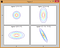Contour plots for 4 different Multivariate Gaussian Distributions. Note that 1st, 2nd and 3rd Gaussian are standard multivariate Gaussian, and 4th is multivariate Gaussian Distribution. Top left Gaussian is referred as 1st Gaussian.

In above figure, note that 1st, 2nd and 3rd Gaussian are axis aligned, while 4th is not. For those who know PCA, if you apply PCA on data generated from 1st, 2nd and 3rd Gaussian, the obtained principle components will be parallel to standard axes(except in case of 1st, because in 1st Gaussian, spread of data is equal in every direction, hence you might get principle components which are non parallel to standard axes), while applying PCA on data generated from 4th Gaussian will give principle components which are non parallel to standard axes.

5. Gaussian Mixture Model: Gaussian Mixture Model(GMM) assumes that data comes from a mixture of multivariate Normal Gaussian, where each Gaussian is assigned a weight. Probability that data is generated from a Gaussian is same as corresponding normalized weight of that Gaussian. Figure 4 may help you understand about GMM.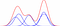Above model has 5 different Gaussian. Actual Probability distribution is shown in Red while underlying Gaussian are shown in Blue. Each Gaussian has a weight which determines it’s contribution in actual probability distribution.

Introduction ends here. Now, let’s dive in the generation process. We’ll first start with Uniform Random Number Generator.

1. Uniform Random Number Generator: Uniform Random Numbers can be generated using Linear Congruential Generators(LCGs). A Linear Congruential Generator is defined by following recurrence relation.

where m is a very large number, 0 < a < m and 0 ≤ c < m. Base value X0 is called seed value. The best choices for a and c are given by Hull-Dobell Theorem, which states that,

i) m and c should be relatively prime. ii) a-1 should be divisible by all prime factors of m. and iii) a-1 is divisible by 4, if 1 is divisible by 4.

However for some cases, any value of a works.

That’s all for Uniform Random Number Generators. Short and Simple. However, there is one problem associated with this. This method generates integers only. But, how to generate real numbers from this. For example, assume that we want to generate a random number in range [0, 1]. How to proceed?

A simple solution to this problem is divide generated random numbers by m. Since every x_i is less than m, we will get a real number between 0 and 1. The precision of generated random number is dependent on m.

Note: Use of LCG is NOT recommended for cryptographic applications. The reason is, even in best case, lower order bit k repeats with period 2^(k+1) i.e. Least significant bit(k = 0) repeats itself after 2 iterations. More secure cryptographically secure pseudo-random number generator is used for these purpose.

2. Normal Random Number Generator: Once a uniform random number is generated, it can be easily transformed into normal random numbers using Box-Muller Transform.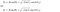Box Muller Transform. Both Polar and Cartesian equations are mentioned. This article discussed only about Cartesian equations. The same numbers can be generated using Polar equations and interestingly, no trigonometric calculations are involved.

This gives two random numbers Z0 and Z1, which are sampled from standard Gaussian distribution.

If you want to generate a random number from a normal distribution having non zero mean and non unit standard deviation, then you can use the transform

X = Z*σ +μ

where X is desired random variable. Let’s see the intuition behind above transform. Assume σ is 1, then

X=Z + μ,

if we see it in terms of graph, it simply means to shift the graph of normal distribution to the right by μ units. Now, let’s tackle the general case,

X = Z*σ + μ.

As we all know, variance captures the spread in the data, so a normal distribution with variance 2 will be more spread than a normal distribution with variance 1. Now, how do we spread a given function. We multiply(or equivalently, divide) the function by some number. And that’s what has been done here. So, in short X = Z*σ + μ, means spread the graph of standard normal distribution by σ units and then shift the distribution in such a way that it’s peak lies at (μ, 0).

3. Standard Multivariate Gaussian Random Vector Generator: Once you have Gaussian random number generator, generating standard multivariate Gaussian Random Vector is a trivial task. As mentioned above in description of Standard Multivariate Gaussian Distribution,

So, in order to generate n-dimensional Standard Multivariate Gaussian random vector, one has to simply generate n i.i.d. random numbers and store them in a list. The list will represent n-dimensional random vector.

Mathematically, relation between independence and uncorrelatedness of standard multivariate Gaussian distribution can be proven just at by looking at the multivariate Gaussian equation.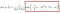Multivariate Gaussian distribution having mean vector μ and co-variance matrix Σ.

Here x is data vector of dimensions 2 by 1, μ is mean vector of dimensions 2 by 1 and Σ is co-variance matrix of dimension 2 by 2.

Note that since every pair of variables are uncorrelated, Σ is a diagonal matrix. Now, the equation can be reduced in following manner:

which can be written as product of two independent Gaussian.Expression standard 2D multivariate Gaussian as product of two Independent Gaussian.

Proving “vice-versa” part is easy, you just have to prove that E(X¹X²) = E(X¹)E(X²), which can be easily proved just by substituting the variables in formula of expectation. This exercise is left for the reader. If you face any problem while doing this, mention it in the comment section.

4. Multivariate Gaussian Random Vector Generator: This is one of the most useful tool in data generation. Most of the times, we know that there is some correlation between the features of the data and we want to sample a n-dimensional random vector from above distribution characterized by mean vector μ and co-variance matrix Σ.

To generate a multivariate Gaussian Random Vector, one has to apply a trick which is similar to inverse PCA. I will publish an article on PCA and inverse PCA by end of next week. For now, just have some faith in me and believe that

Y = P * sqrt(Λ) * X

Here:

Y —multivariate Gaussian random vector.

P — matrix containing eigenvectors of Σ, sorted by eigenvalues.

Λ— Diagonal matrix containing eigenvalues of Σ in descending order.

X — standard Multivariate Gaussian Random Vector sampled from a standard multivariate Gaussian distribution having mean vector μ.

This gives a multivariate Gaussian random vector sampled from a multivariate Gaussian distribution having mean vector μ and co-variance Σ.

5. Data generation from Gaussian Mixture Model:- Finally, coming to most the useful model in data generation. As mentioned in the introduction, GMM models the data using mixture of Gaussian having mean vector μ and co-variance vector Σ. Each Gaussian in the mixture has it’s a weight attached to it. Once you understood above lines, then generating a random vector from GMM is a trivial task. Here are the steps for the same:

a. Normalize the weights of each Gaussian distribution such that sum of all normalized weights is equal to 1.

b. After normalization, it can be assumed that probability of selection of Gaussian from which data has to be generated is same as it’s corresponding normalized weight.

c. Once assumption has made, a PDF for weights can be obtained.

d. Construct a CDF for the weights. A sample CDF may look like

e. Once CDF is obtained, generate a uniform random number using uniform random number discussed above. Perform a lookup on CDF to determine which probability distribution to select.

f. Once the Gaussian is fixed, sample a random number from that Gaussian using normal random number generator or multivariate random vector generator discussed above.

This completes the discussion on data generation from different types of Gaussian distributions. I will soon write an article covering other distributions. I tried to keep this article more intuitive and less mathematical. If you have any issues/suggestions, kindly comment.

Code for generating random numbers/vectors from all above discussed distributions can be found here.

Written by